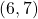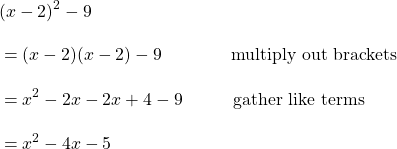Let’s plot the points on the graph that satisfy. That is, for each value ofplot.

Every quadratic function of the formhas this same shape. However, the coefficientsandalter the position and steepness of the parabola. Try different values ofandon this applet.

# What’s a parabola good for?

1.  A projectile. The height above the ground at time after launch (of a rocket, basket ball, slingball, or any other object thrown or dropped) can be modelled with a parabola.https://dewwool.com/20-examples-of-projectile-motion/

2. Design (Engineering) and Animation (Films). Pixar partnered with Khan Academy to produce the ‘Pixar in a Box’ series of lessons and tutorials. Where math meets visual and performing arts.pixarinabox

3. This prezi showcases some parabola in action and offers a summary of the whole unit.

# Three Forms of a Quadratic Expression

A quadratic expression has three common presentations.

1. General form:2. Factored form3. Completed square (or vertex) formLet’s see the expressionin these three forms:

1. General form2. Factored form3. Completed square formThese three forms are equivalent. No matter what value ofyou might choose, the three forms will compute to the same value. Let’s check with1.2.3.Here is the graph of the function. Note the pointlies on the parabola.In this unit, we find out

• how to graph a parabola beginning with any of the three forms;
• how to algebraically convert from one form of quadratic expression to another;
• how to solve a quadratic equation.

# Multiplying out Brackets

It is assumed that you know how to multiply out brackets such as. If not, you can review multiplying polynomials here.

By multiplying out the brackets we can already convert from factored form to general form:We can also convert from completed square form to general form:Check out these pages for the reverse operations: